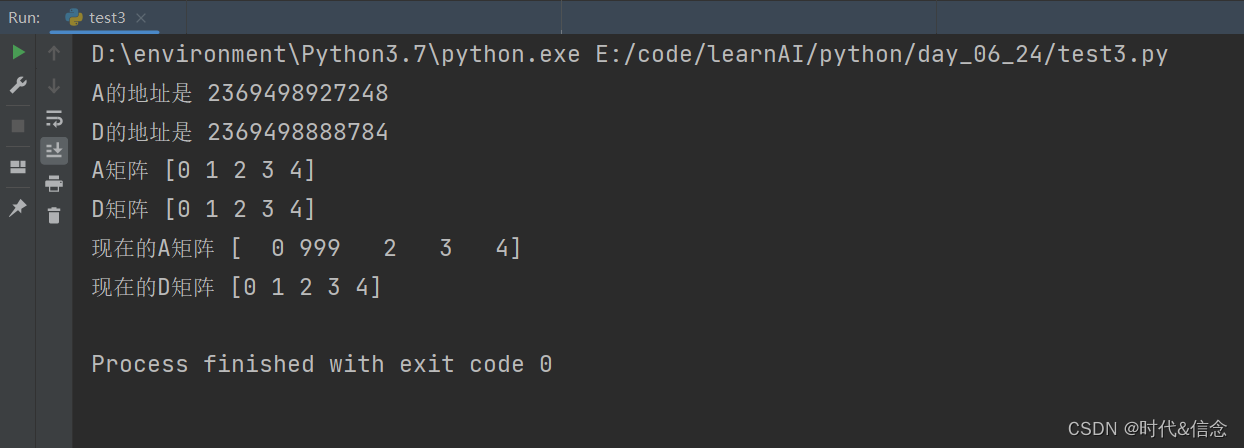# 用于 Python 数据分析的 Numpy（保姆级教程）

## 二、前提准备

（1）Win + R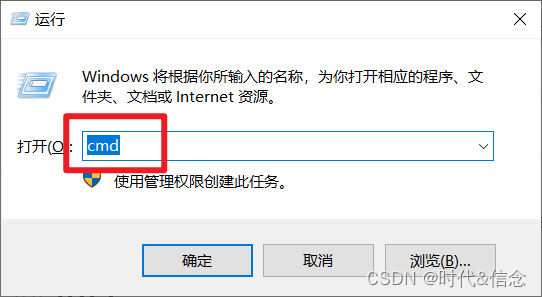（2）然后在Windows终端中输入如下命令，回车即可。

``````pip install numpy
``````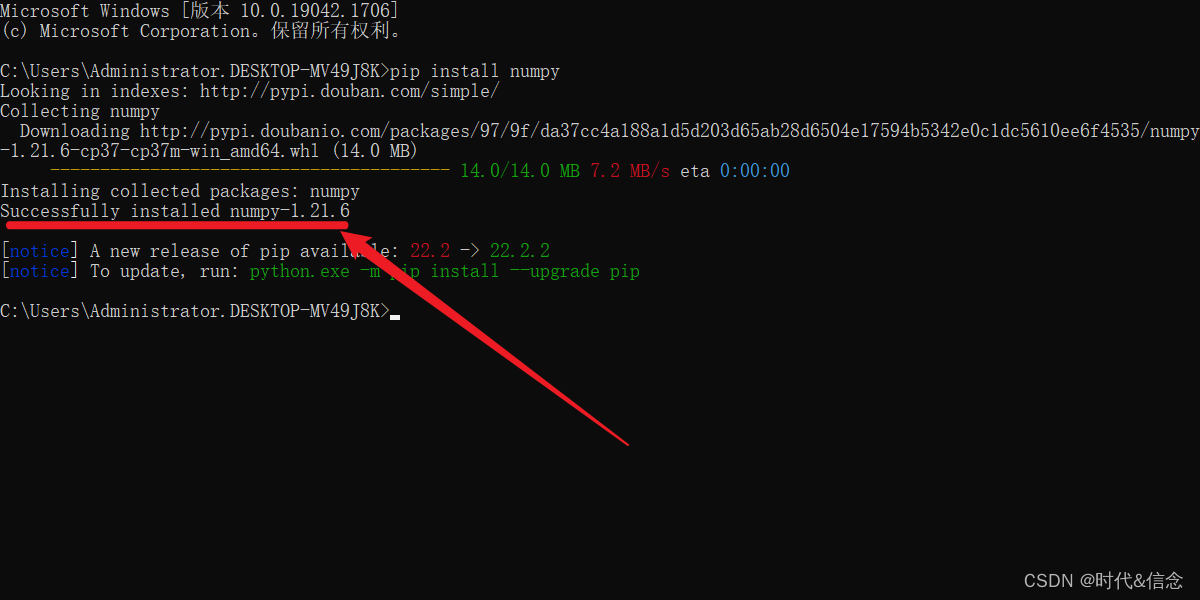## 三、具体使用

### 1.numpy的属性

``````# coding:utf-8
import numpy as np
"""
numpy的属性
"""

# 将列表转化成一个numpy的矩阵
array = np.array([[1, 2, 3],
[4, 5, 6]])
# 输出矩阵
print(array)
# 输出矩阵的维数
print("dim", array.ndim)
# 输出矩阵形状
print("shape", array.shape)
# 输出矩阵尺寸（元素总个数）
print("size", array.size)
``````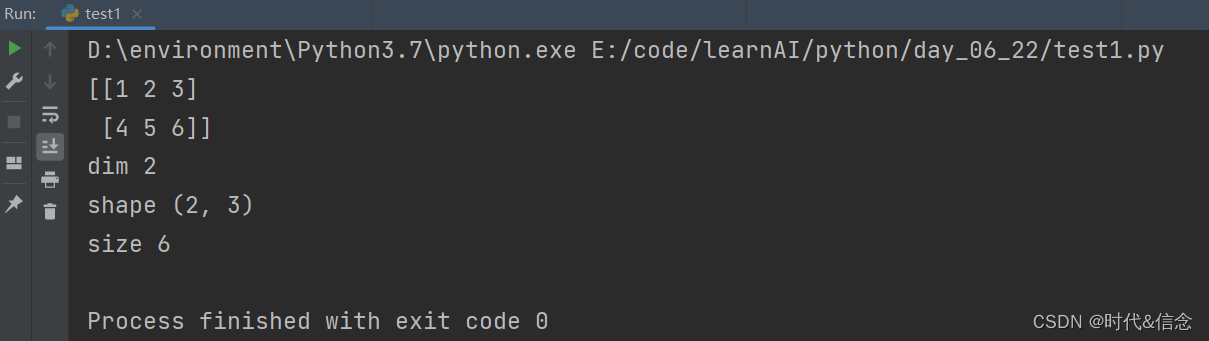### 2.numpy中的创建array

#### （1）矩阵和列表的区别

``````# coding:utf-8
import numpy as np

"""
输出矩阵的各种形式！！！！
"""

#   矩阵输出出来，中间没有逗号；
#     而列表打印出来会有逗号
a_list = [1, 2, 3]
print(a_list)
a_np_array = np.array([1, 2, 3])
print(a_np_array)
``````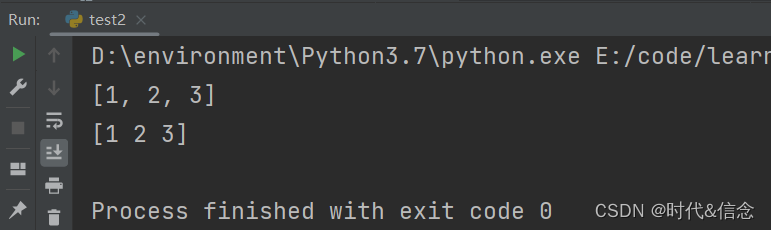#### （2）设定矩阵中数值的类型

``````# dtype是用来设定矩阵中数值的数据类型
a = np.array([[1, 2, 3], [4, 5, 6]], dtype=np.int64)
print(a.dtype)
print(a)
``````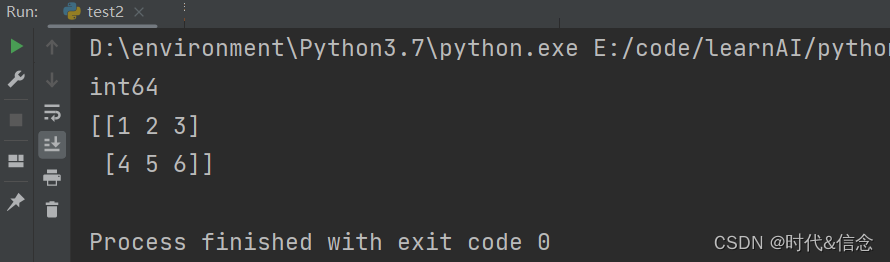#### （3）生成规定形状的、元素都为0的矩阵

``````# 生成3行4列的零矩阵
b = np.zeros((3, 4))
print(b)
``````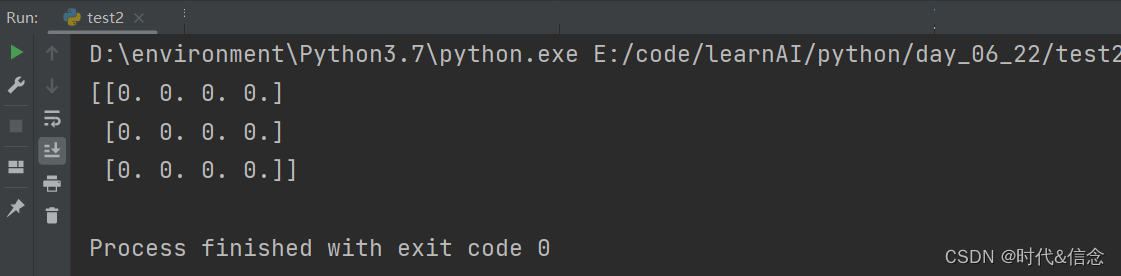#### （4）生成规定形状的、元素都为1的矩阵

``````# 生成数值全部是1的矩阵
c = np.ones((2,4), dtype=np.int32)
print(c)
``````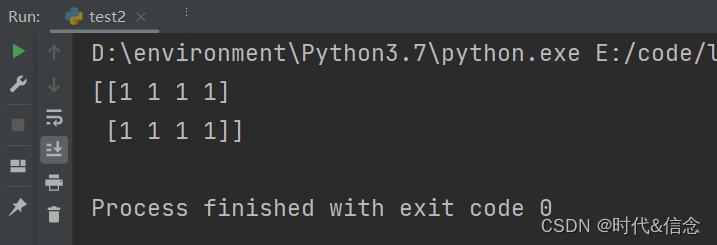#### （5）生成规定形状的、元素都为empty（实际元素都接近于0）的矩阵

``````# # 输出empty的矩阵
d = np.empty((3,4))
print(d)
``````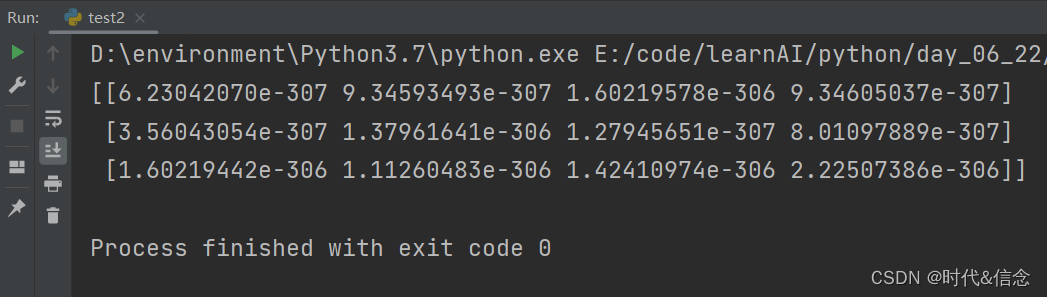#### （6）生成顺序数值的矩阵

``````# 输出顺序的矩阵,生成数据类比range; 从10到20，左闭右开，步长为2
e = np.arange(10, 20, 2)
print(e)
``````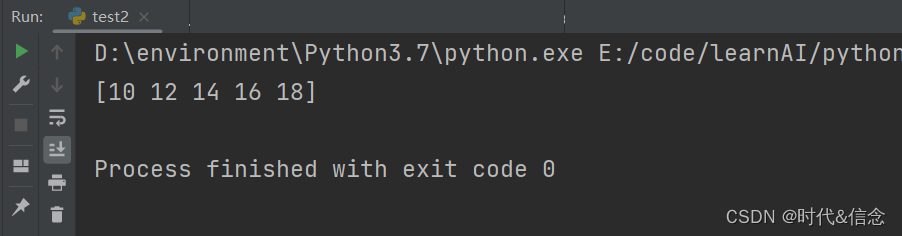#### （7）修改矩阵形状

``````# 输出顺序的矩阵,按顺序以三行四列的格式进行输出
f = np.arange(12).reshape(3, 4)
print(f)
``````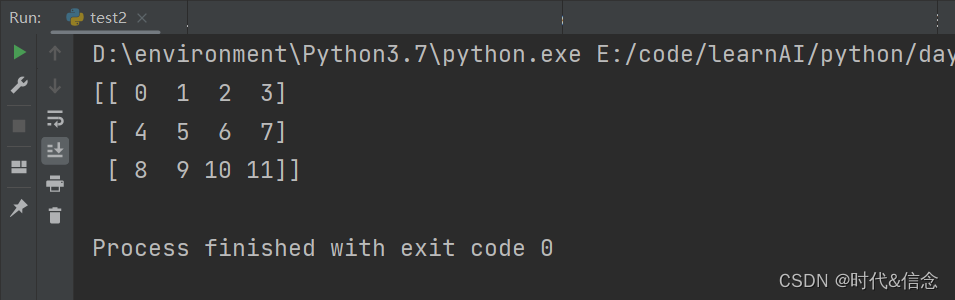#### （8）按规定生成“线段”数值的矩阵

``````# 输出线段,1是起始值，10是终止值，6是生成数值个数，从1到10生成6个数，并且他们之间是等距的
# 自动匹配步长
g = np.linspace(1, 10, 6).reshape(2, 3)
print(g)
``````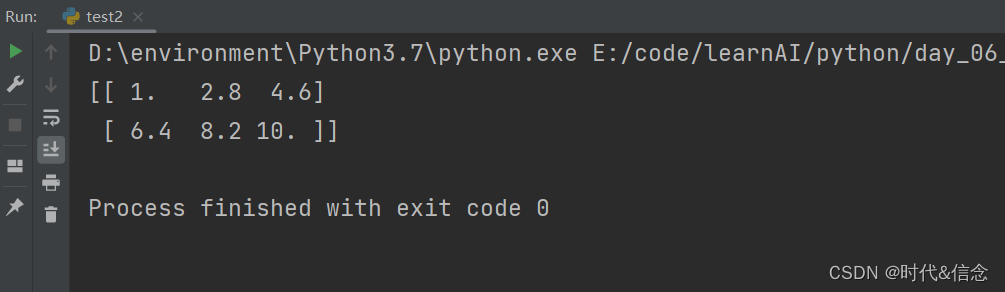### 3.numpy的基础运算

#### （1）矩阵减法（对应元素相减）

``````# coding:utf-8
import random

import numpy as np
"""
numpy的基础运算
"""

a = np.array([10, 20, 30, 40])
b = np.arange(4)
print("a矩阵", a)
print("b矩阵", b)
# 矩阵减法
c = a - b
print("c矩阵", c)
``````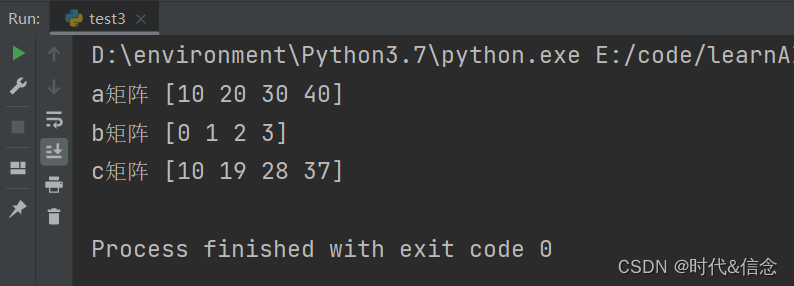#### （2）矩阵加法（对应元素相加）

``````# 矩阵加法
d = a + b
print("d矩阵", d)
``````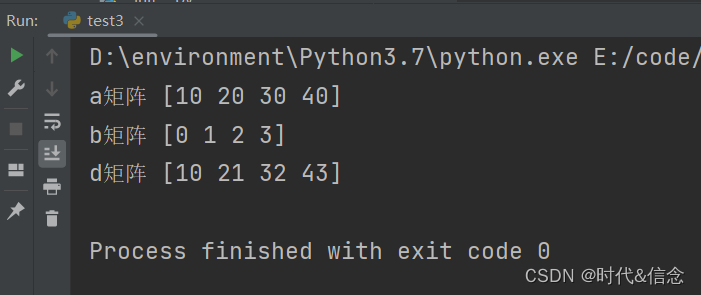#### （3）矩阵数值平方运算

``````# 进行平方
e = b**2
print("e矩阵", e)
``````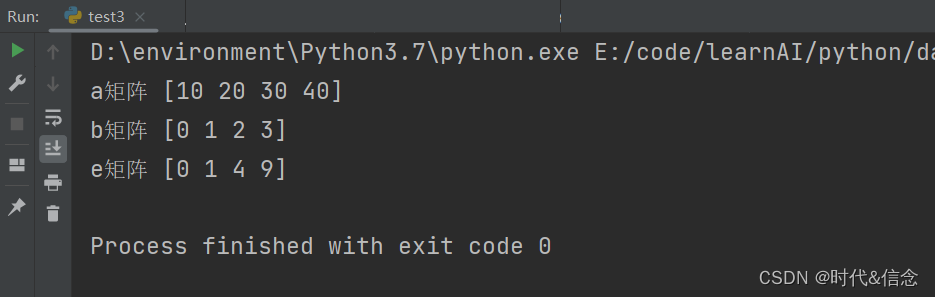#### （4）矩阵各个元素求三角函数

``````# 调用numpy中的三角函数, sin ,cos ,tan
f = np.sin(a)
print(f)
``````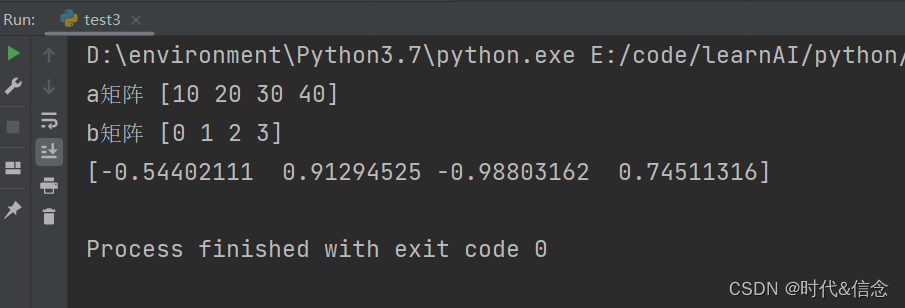#### （5）判断矩阵中哪些值是小于某个数的

``````# 判断矩阵中哪些值是小于3的
# 小于号可以换成其他，比如==，>
print(b)
print(b<3)
``````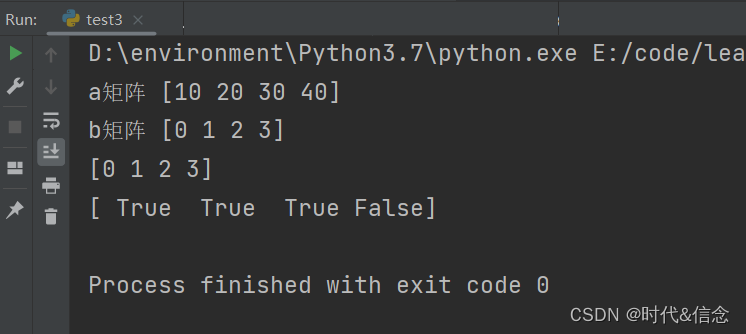#### （6）矩阵之间数值逐个相乘

``````g = np.array([[1, 1],
[0, 1]])
h = np.arange(4).reshape((2,2))

print(g)
print(h)

# 每个元素挨个相乘
i = g * h
print(i)
``````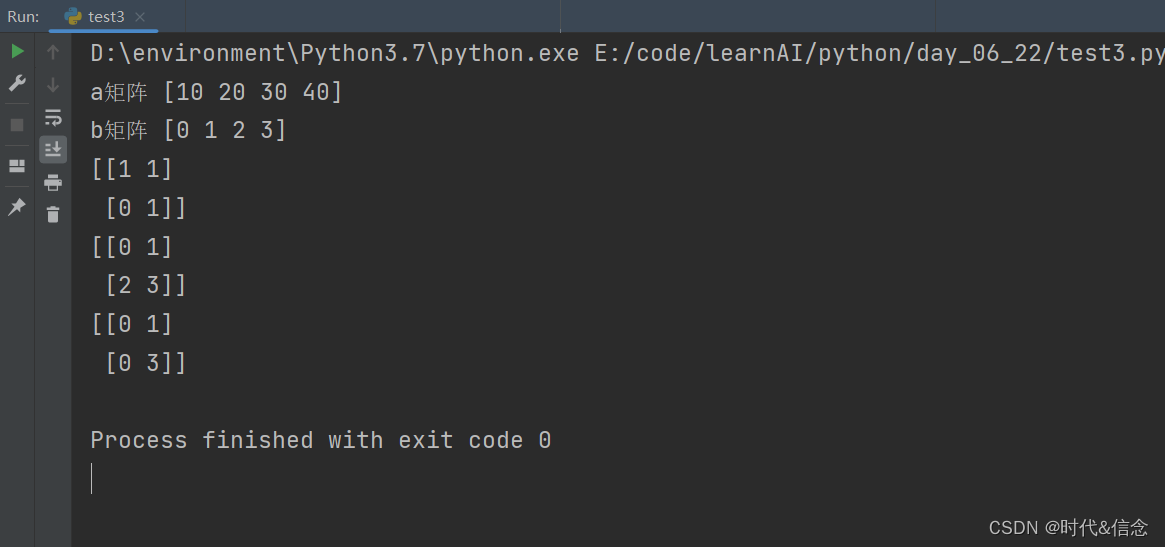#### （6）矩阵乘（线性代数中的矩阵乘）

``````# 矩阵相乘
j = np.dot(g, h)
print(j)

# 矩阵相乘的另一种形式
k = g.dot(h)
print(k)

``````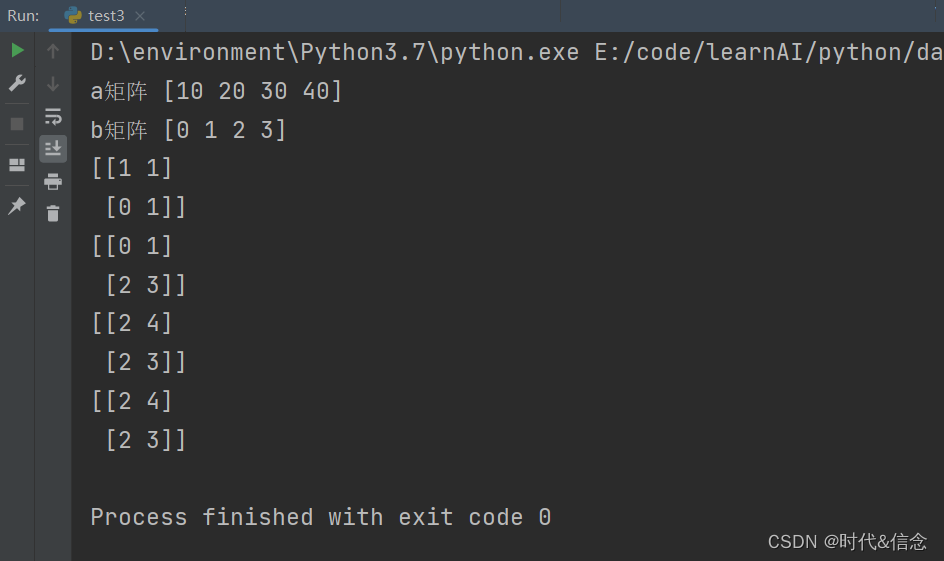#### （7）生成规定形状的随机数矩阵

``````# 生成随机数矩阵,(2,4)表示矩阵形状，元素值的范围是0~1
l = np.random.random((2,4))
print(l)
``````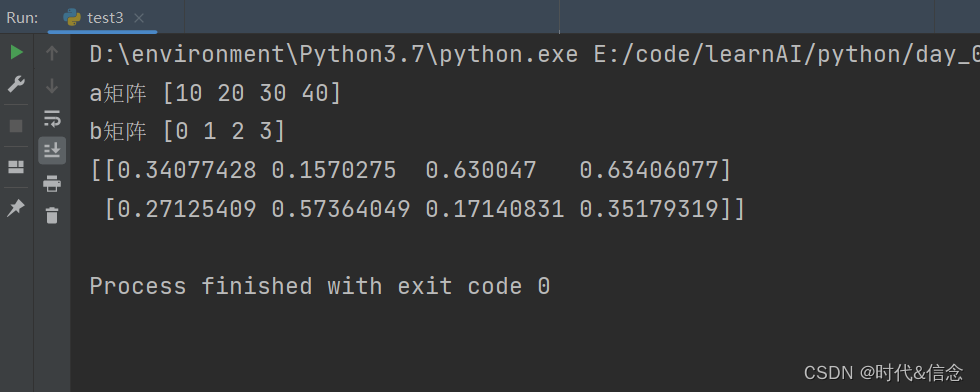#### （8）矩阵中所有元素求和、求最大值、求最小值

``````# 对矩阵中的数值进行求和、求最大值、求最小值
# 矩阵中所有元素进行求和
print(np.sum(l))
# 矩阵中的最大值
print(np.max(l))
# 矩阵中的最小值
print(np.min(l))
``````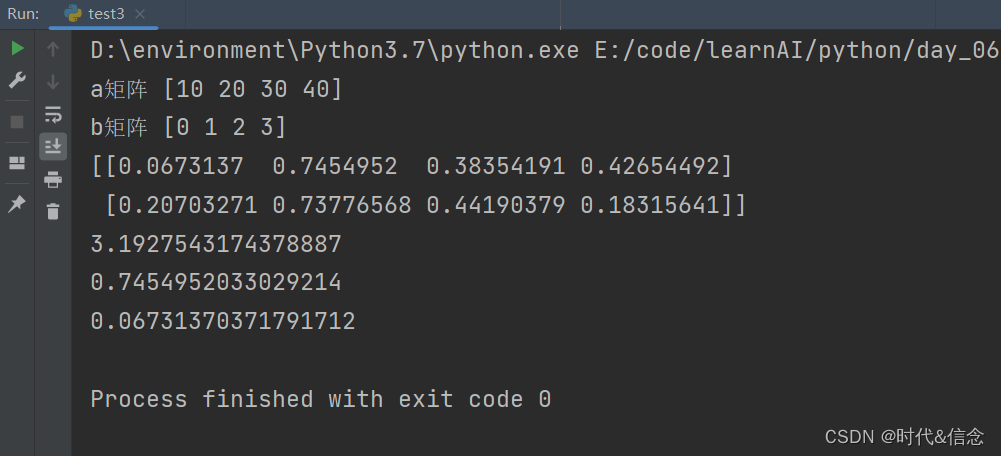#### （9）对矩阵中指定行和列进行求和、求最大值、求最小值

``````# 对指定行和列进行求和、求最大值、求最小值
# 其中axis参数值为0时，代表列；参数值为1时，代表行
print(np.sum(l, axis=1))
print(np.max(l, axis=0))
print(np.min(l, axis=1))
``````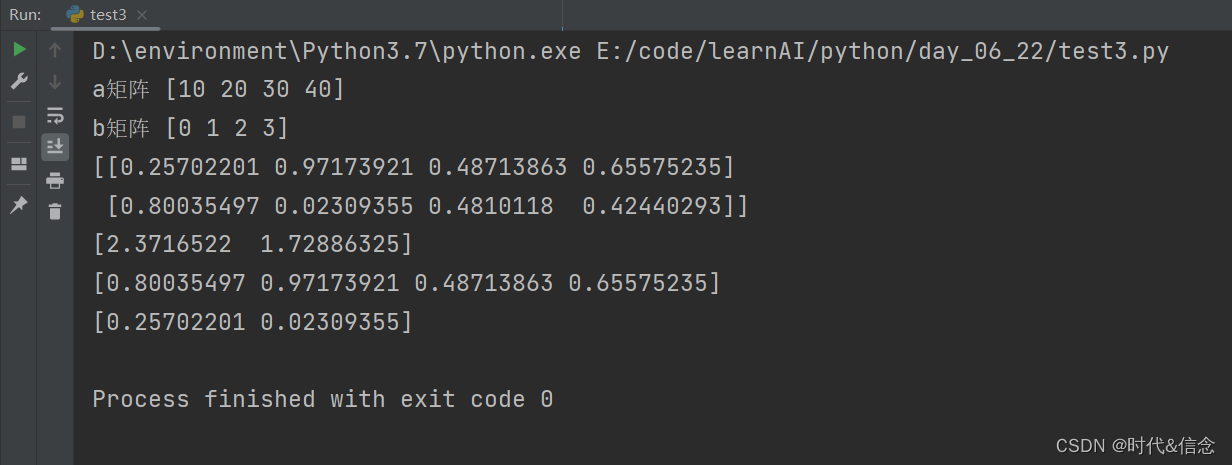#### （10）求矩阵中最小值和最大值的索引

``````# coding:utf-8
import numpy as np
"""
numpy中的运算
"""

# 生成顺序数值，并设定为三行四列
A = np.arange(2, 14).reshape(3, 4)
print(A)
# 输出矩阵中数值最小的那个值所在的索引
print(np.argmin(A))
# 最大值对应的索引
print(np.argmax(A))
``````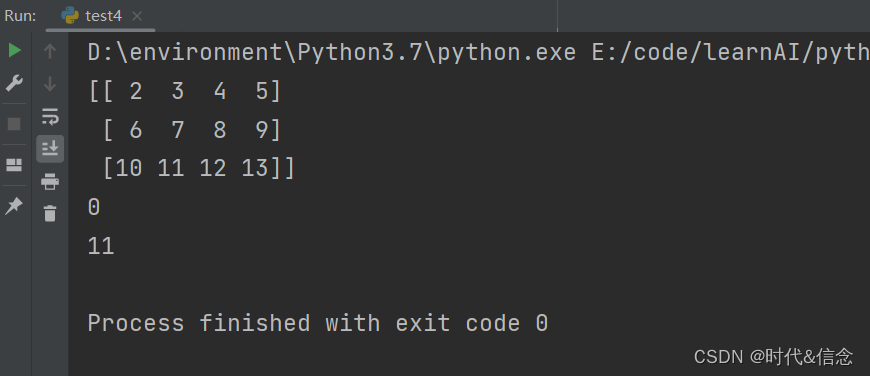#### （11）求矩阵中元素的平均数和中位数

``````# 输出矩阵中的平均数
print(np.mean(A))

# 另外几种形式
print(A.mean())
print(np.average(A))
# 输出矩阵中的中位数
print(np.median(A))
``````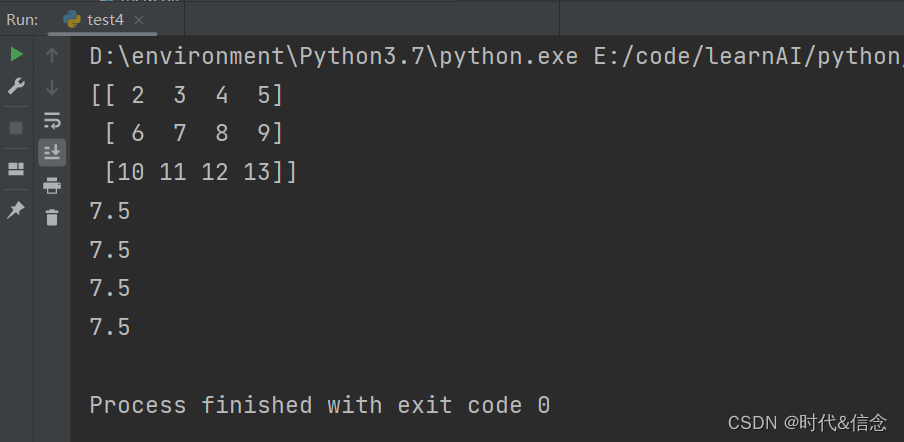#### （12）矩阵中前几项的累计

``````# 矩阵中前几项的累计
print(np.cumsum(A))
``````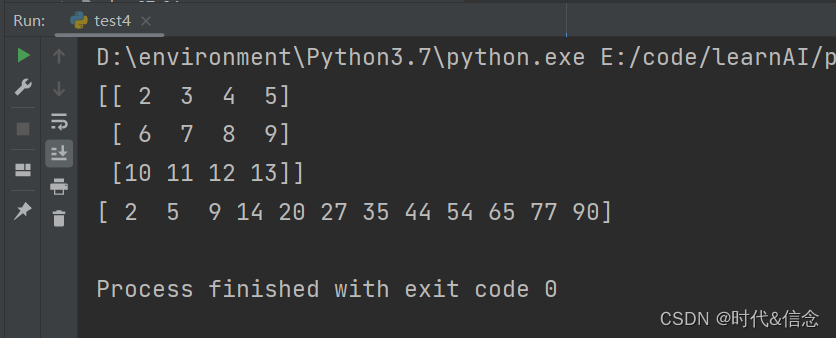#### （13）矩阵中元素后一项与前一项的差值

``````# 矩阵数值之间，每两个数值之间的差值
print(np.diff(A))
``````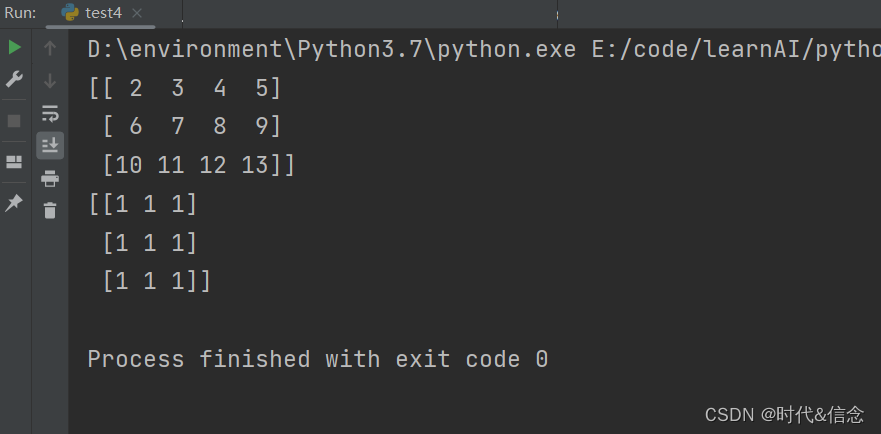#### （14）以行数组和列数组，输出矩阵中非0数值

``````# 以行数组和列数组，输出矩阵中非0数值
print(np.nonzero(A))
``````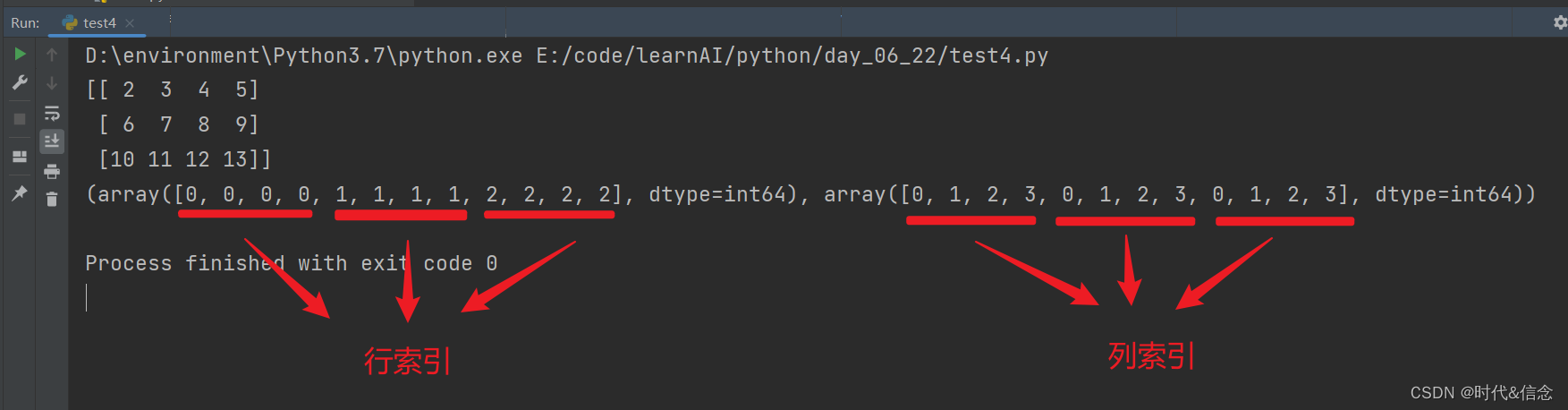#### （15）对矩阵进行逐行排序

``````B = np.array([[5, 9, 2],
[1, 0, 7]])
print(B)
# 使用sort对矩阵数值进行 逐行排序
print(np.sort(B))
``````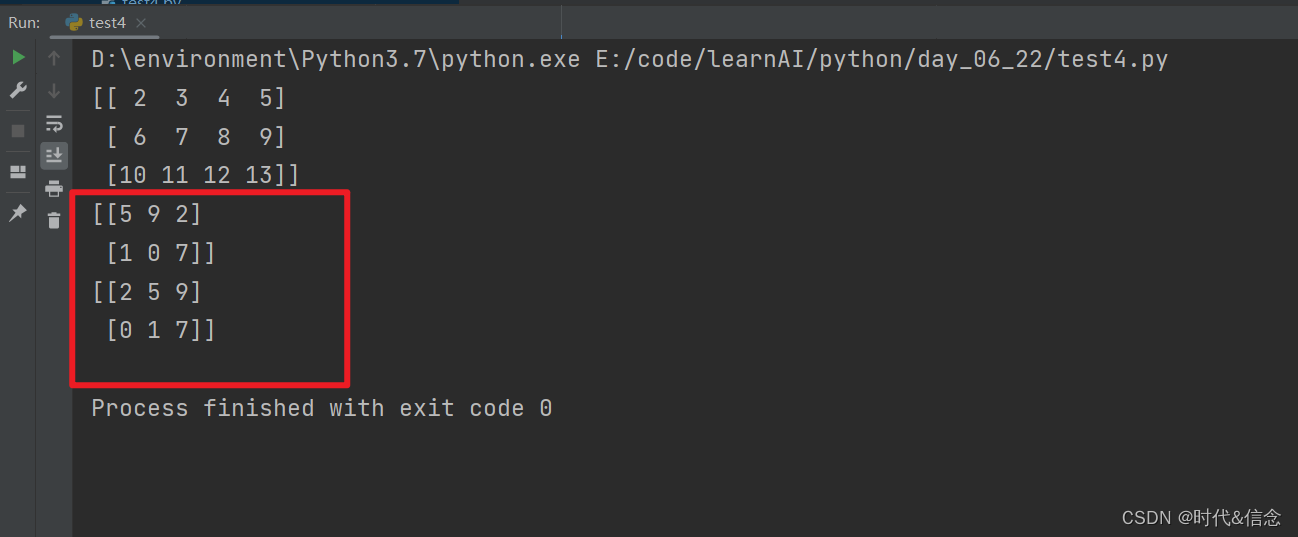#### （16）对矩阵进行转置

``````# 对矩阵进行转置
print(np.transpose(A))
print(A.T)
``````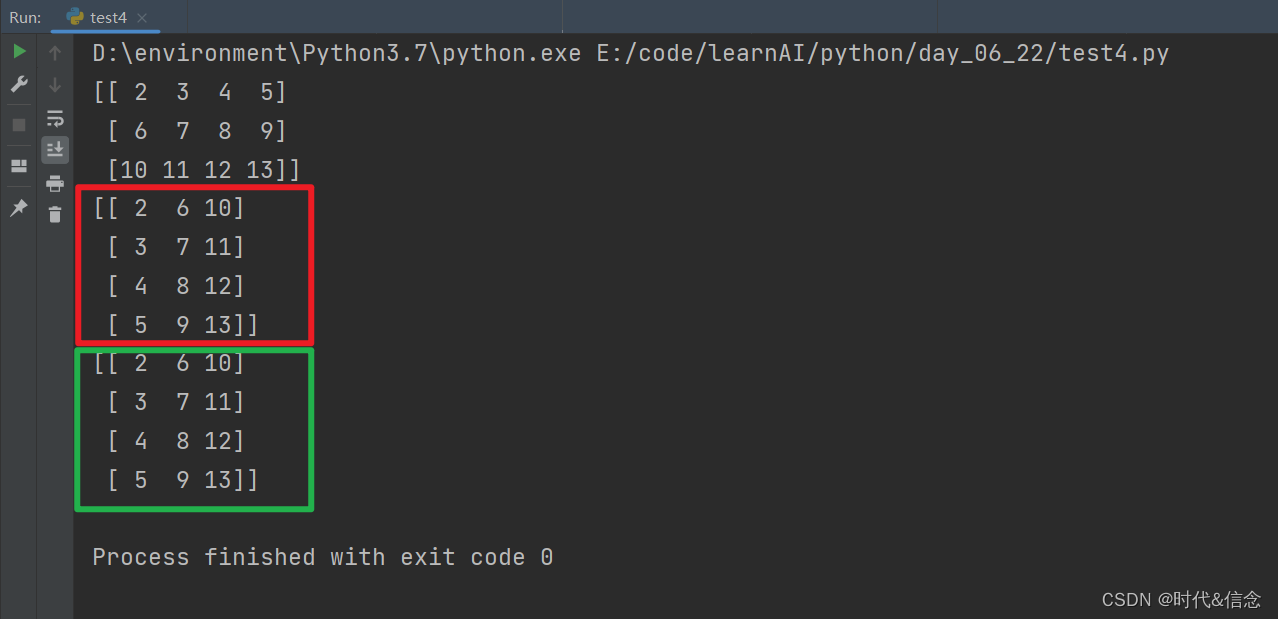#### （17）矩阵数值的“截断”

``````# 矩阵中小于5的值全部变成5；大于9的值全部变成9，位于5到9之间的数值，保持不变
print(np.clip(A, 5, 9))
``````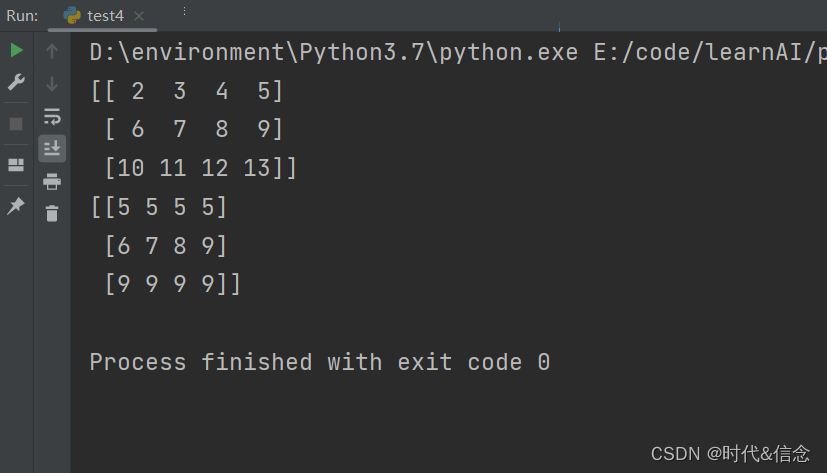#### （18）对矩阵中行元素求平均、对列元素求平均

``````# 对行算平均值，对列算平均值
# 参数为0是对行算平均值，参数为1是对列算平均值
print(np.mean(A, axis=0)) # 对列
print(np.mean(A, axis=1)) # 对行
``````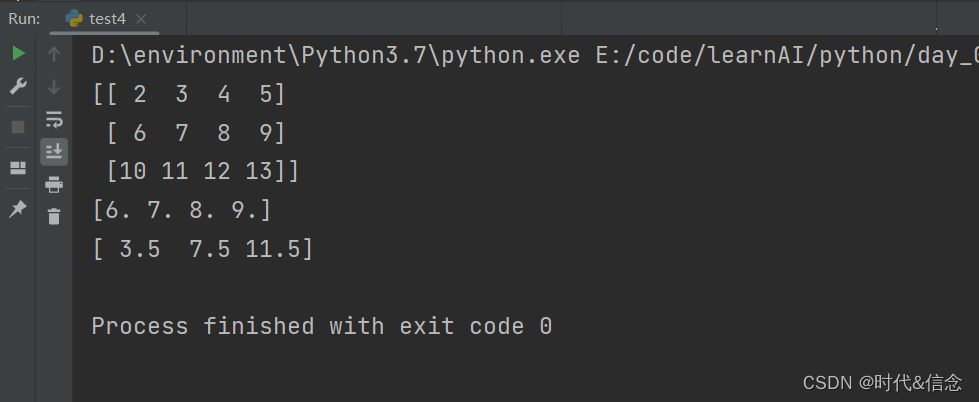### 4.numpy的索引

#### （1）通过索引输出矩阵中的对应值

``````# coding:utf-8
import numpy as np
"""
numpy中的索引
"""
A = np.arange(3, 15).reshape((3, 4))
print(A)
# 矩阵中，指定索引的值
print(A)  # [11, 12, 13, 14]

# 输出指定索引对应的数值
print(A) # 8
# 也可以是这种形式进行具体索引的输出
print(A[1, 1]) # 8
``````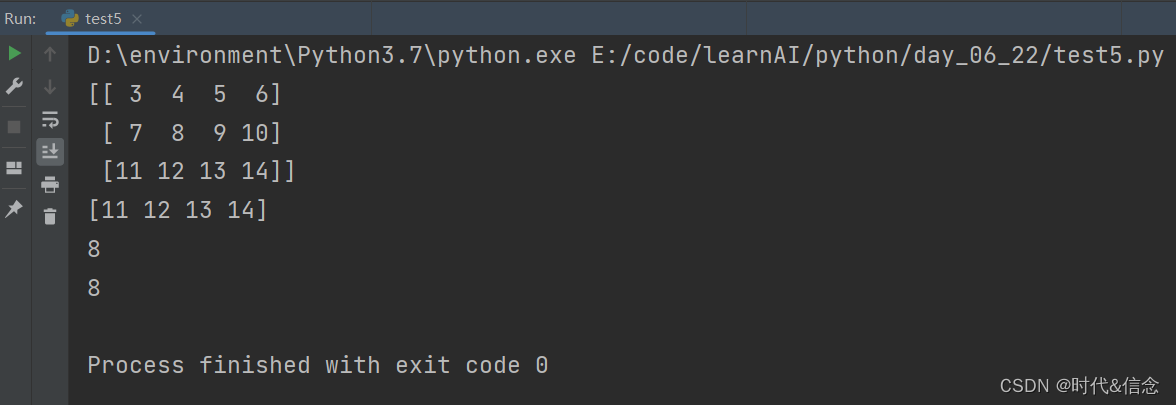#### （2）矩阵中的“切片”

``````# 打印第二行的所有数
print(A[2, :])
# 打印第一列的所有数
print(A[:, 1])

# 在python中满足左闭右开的原则
print(A[1, 1:3])
``````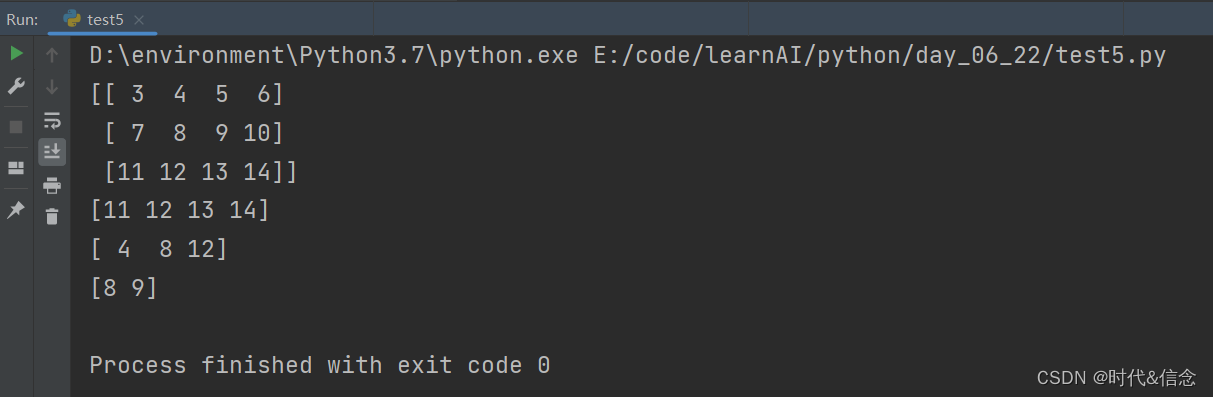#### （3）迭代矩阵的每一行

``````# 循环起来，打印行
print("#" * 20)
for row in A:
print(row)
``````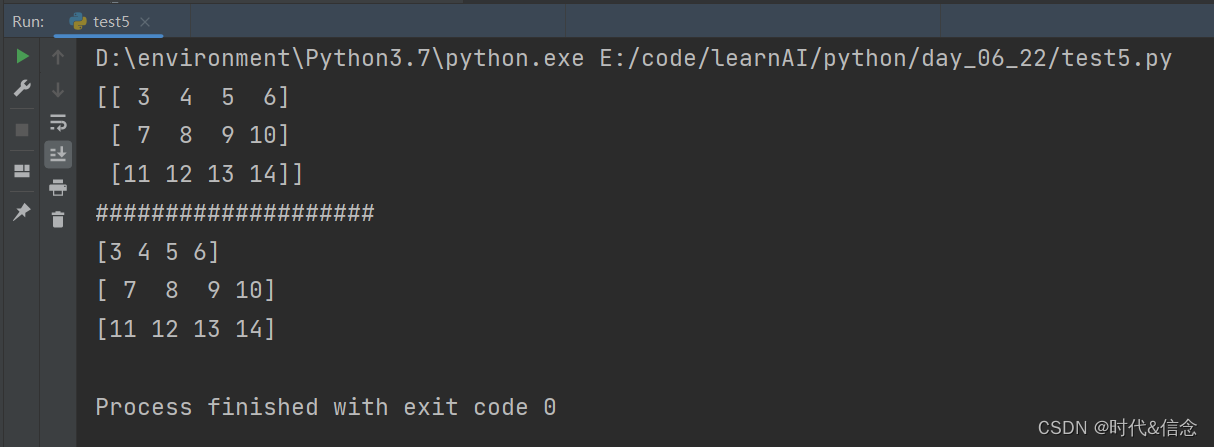#### （4）迭代矩阵中的每一列

``````# 打印列
for column in A.T:
print(column)
``````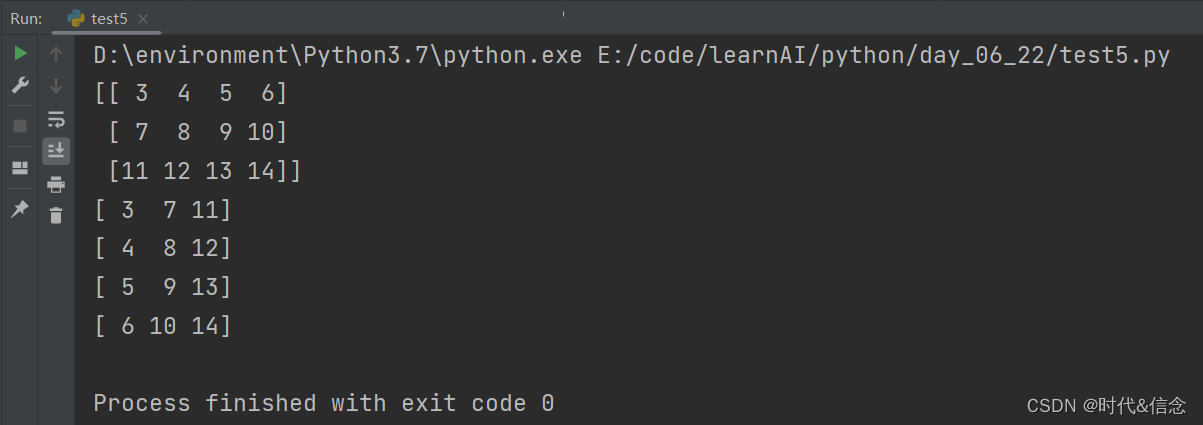#### （5）迭代矩阵中的每一项数值（先将矩阵拉成只有一行的矩阵）

``````# 将矩阵拉直为一维矩阵
print(A.flatten())
# 矩阵
print(type(A.flatten()))
# 想要迭代每一个元素，需要这样
print("迭代每一个数值")
for item in A.flat:
print(item)
``````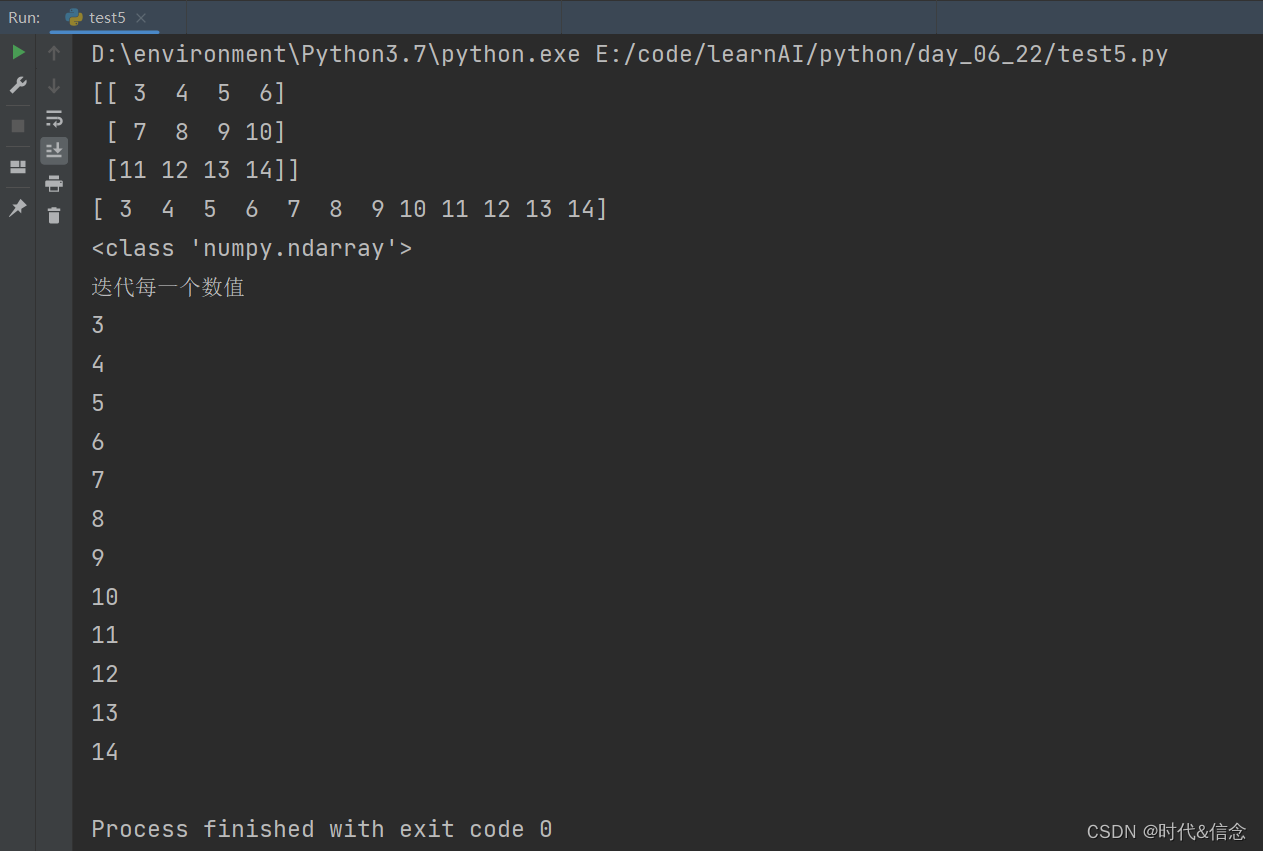### 5.numpy中array的合并

#### （1）两个矩阵，竖直方向上的合并

``````# coding:utf-8
import numpy as np
"""
numpy中的合并
"""

# 竖直方向上的合并
A = np.array([1, 2, 3])
B = np.array([1, 1, 1])
print(np.vstack((A, B)))
# 输出矩阵形状
print(np.vstack((A, B)).shape)
``````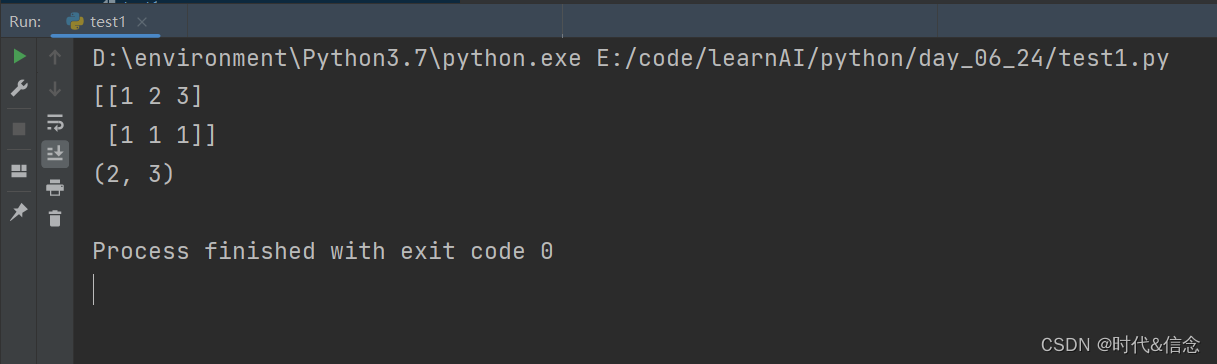#### （2）两个矩阵，水平方向上的合并

``````# 水平方向上的合并
print(np.hstack((A, B)))
print(np.hstack((A, B)).shape) # (6,)表示一行六列的矩阵

print("原来的A矩阵", A)
# 输出矩阵A的形状
print(A.shape)  # (3,)表示一行三列的矩阵
``````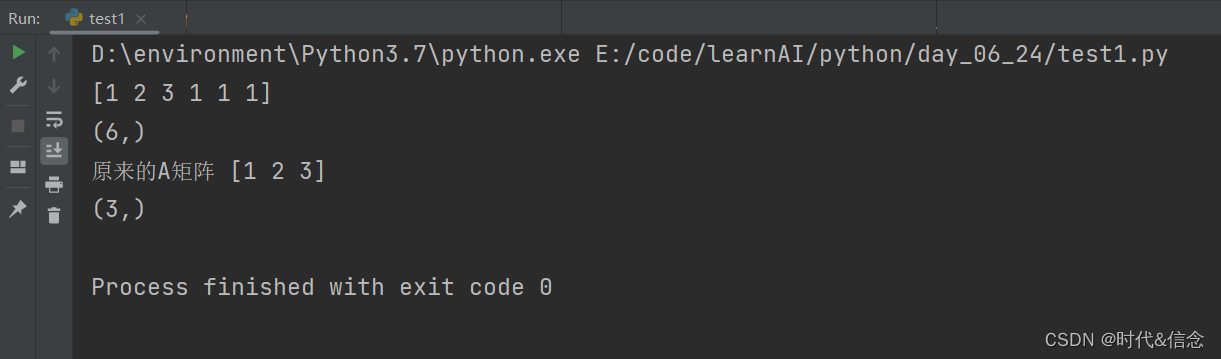#### （3）给矩阵加维度

``````print("原来的矩阵", A)
# 给列加上维度
print(A[:, np.newaxis])
# 给行加上维度
print(A[np.newaxis, :])
``````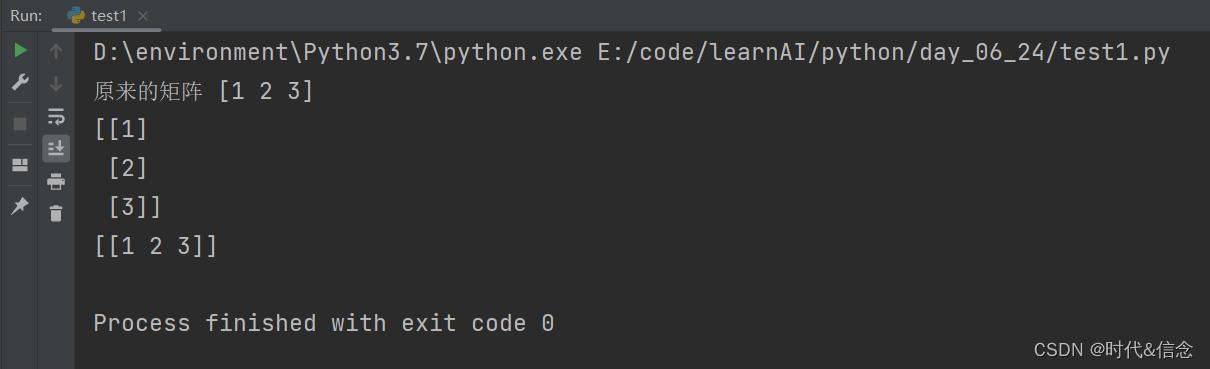#### （4）添加维度之后进行矩阵合并

``````# 当矩阵为1行是就默认为列表形式了，转置之后也是列表形式
# 比如说一行三列，转置之后仍然是一行三列
# 要想将一行三列变成三行一列，应该使用：加维度
print(A)
print(A.T)
A = np.array([1, 2, 3])[:, np.newaxis]

print(A)
print(type(A))
print(A.shape)
B = np.array([1, 1, 1])[:, np.newaxis]

C = np.hstack((A, B))
print("水平合并之后")
print(C)
D = np.vstack((A, B))
print("竖直合并之后")
print(D)
``````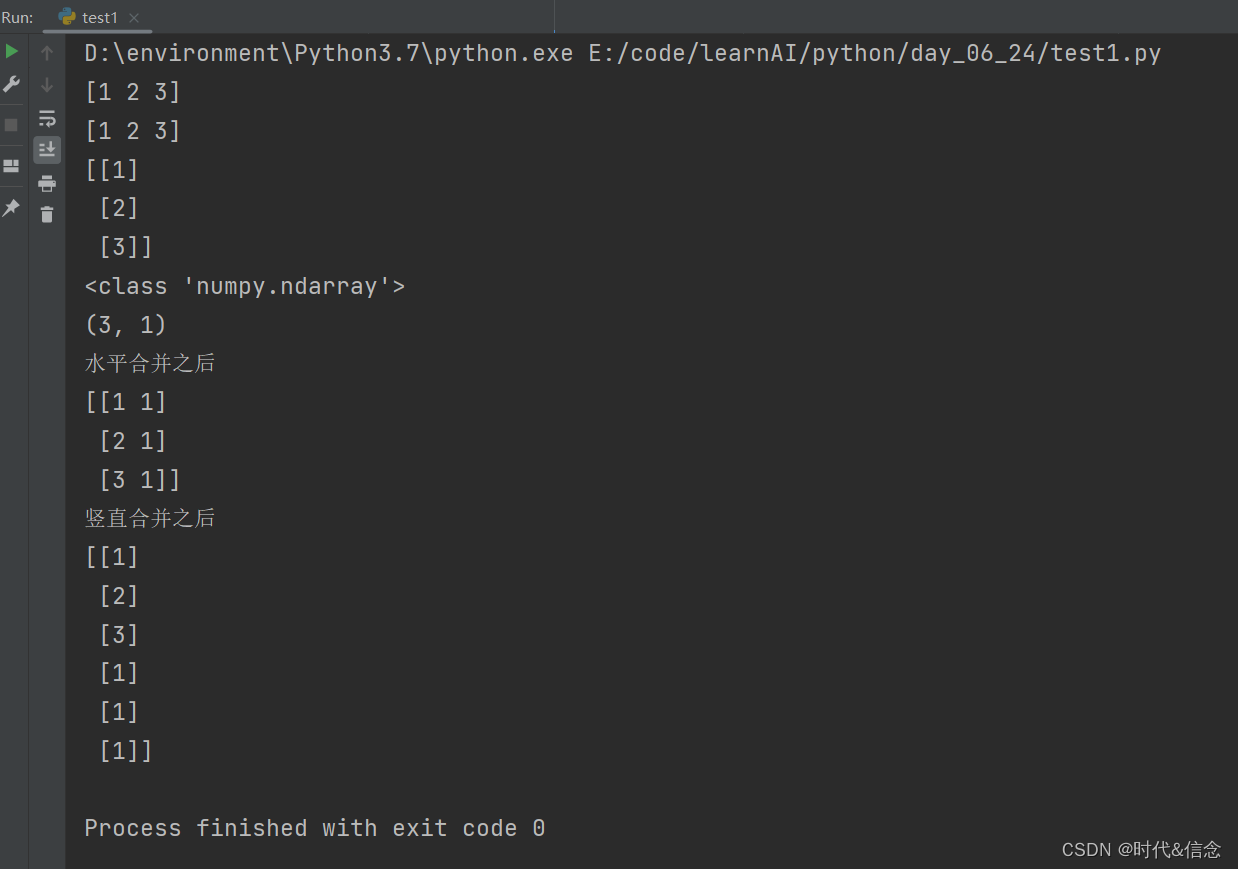#### （5）多个矩阵的合并（自己设定竖直方向还是水平方向）

``````# 多个矩阵进行合并,竖直方向合并, 0代表竖直方向， 1代表水平方向
A = np.array([1, 2, 3])[:, np.newaxis]
B = np.array([1, 1, 1])[:, np.newaxis]
E = np.concatenate((A, B, A, A), axis=0)
F = np.concatenate((A, B, A, B), axis=1)
print("-" * 20)
print(E)
print("&" * 20)
print(F)
``````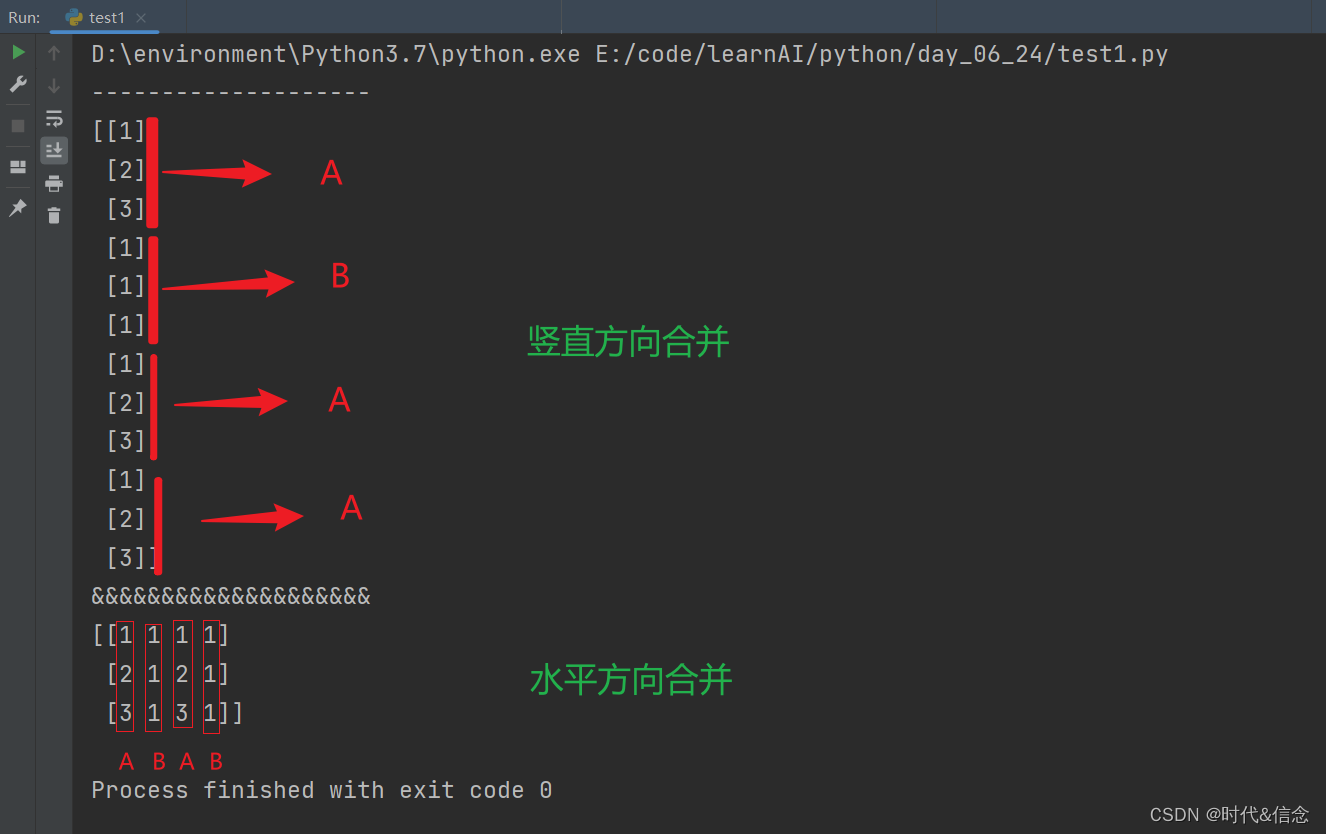### 6.numpy中array的分割

#### （1）进行等分

``````# coding:utf-8
import numpy as np
"""
分割矩阵
"""

# 生成一个三行四列的矩阵
A = np.arange(12).reshape((3, 4))
print(A)

# 对矩阵进行分割
# 第一个参数代表被分割的矩阵，第二个参数代表分割的块数，第三个参数代表分割的方向
# 0代表竖直分割，1代表水平分割
print(np.split(A, 2, axis=1))

# 纵向分割成三块
print(np.split(A, 3, axis=0))
``````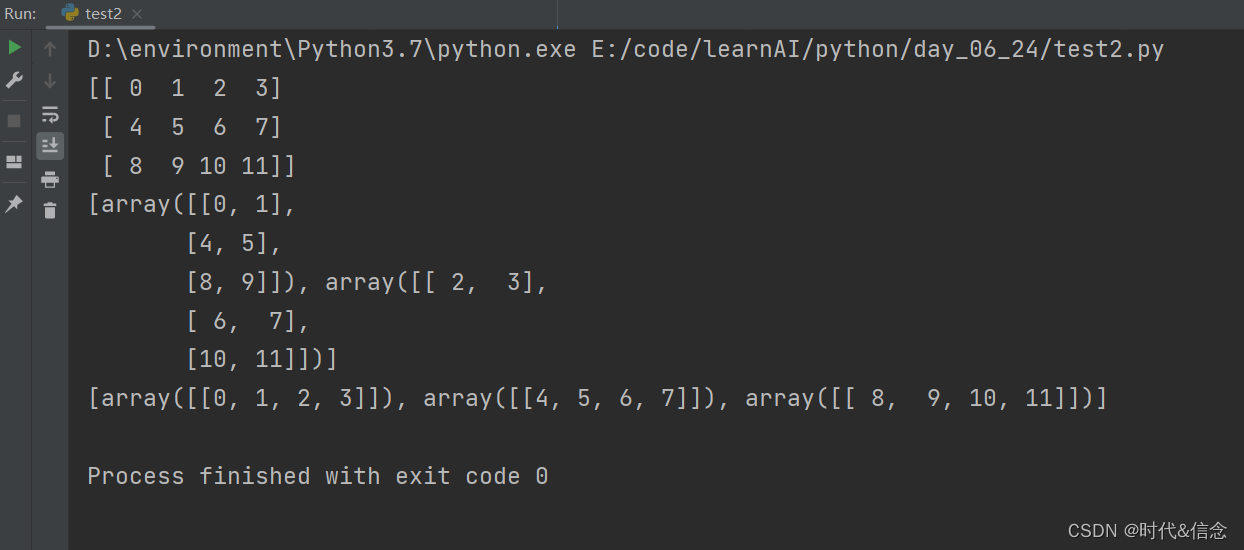#### （2）进行不等分

``````# split只能分割成相等的，不能不等分

# 使用array_split进行不等分割
print(np.array_split(A, 3, axis=1))
``````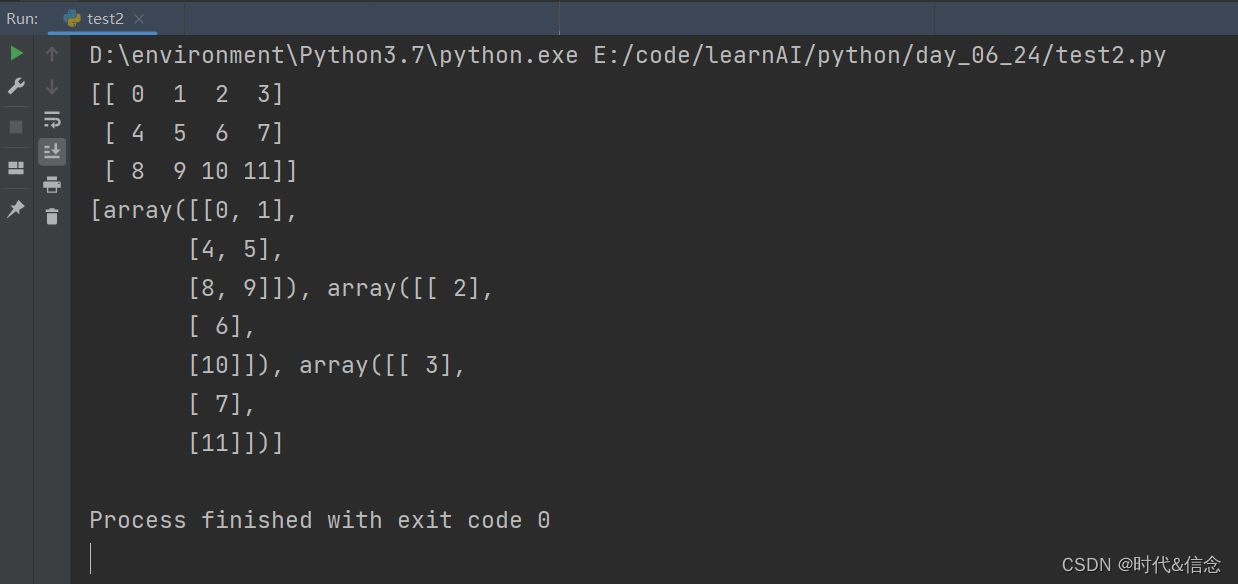#### （3）另一种方式的分割函数的使用

``````# 横向纵向指的是最后如何排列的
# 或者这样理解，垂直切是在垂直方向上切的；水平切是在水平方向上切的
# 直接使用函数进行纵向分割
print("纵向分割---------------------------")
print(np.vsplit(A, 3))
# 直接使用函数进行横向分割
print("横向分割---------------------------")
print(np.hsplit(A, 2))
``````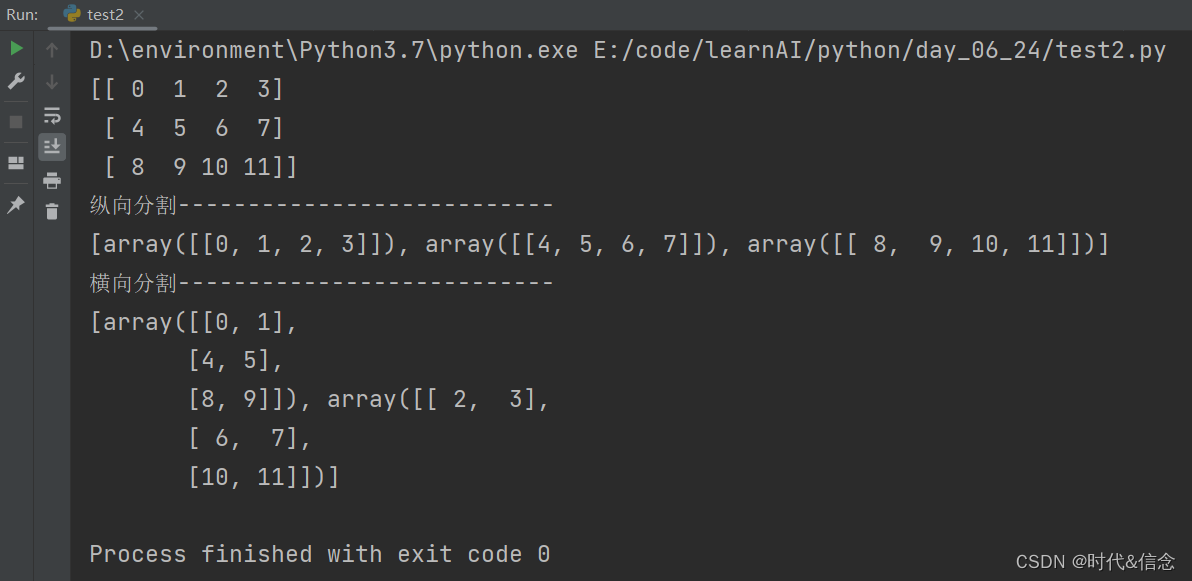### 7. numpy中的深拷贝和浅拷贝

#### （1）矩阵赋值（浅拷贝）

``````# coding:utf-8
import numpy as np

"""
numpy矩阵中的深拷贝和浅拷贝
"""

# 赋值，其实就还是公用一片内存
A = np.arange(5)
print("A矩阵", A)
B = A
C = A
print("B矩阵", B)
print("C矩阵", C)

# 改变A中数值，因为地址相同，所以其他浅拷贝之后的矩阵也都会发生改变
A = 666
print("现在的A矩阵", A)
print("现在的B矩阵", B)
print("现在的C矩阵", C)

# 赋值是浅拷贝，地址其实一致
print(id(A))
print(id(B))
print(id(C))
``````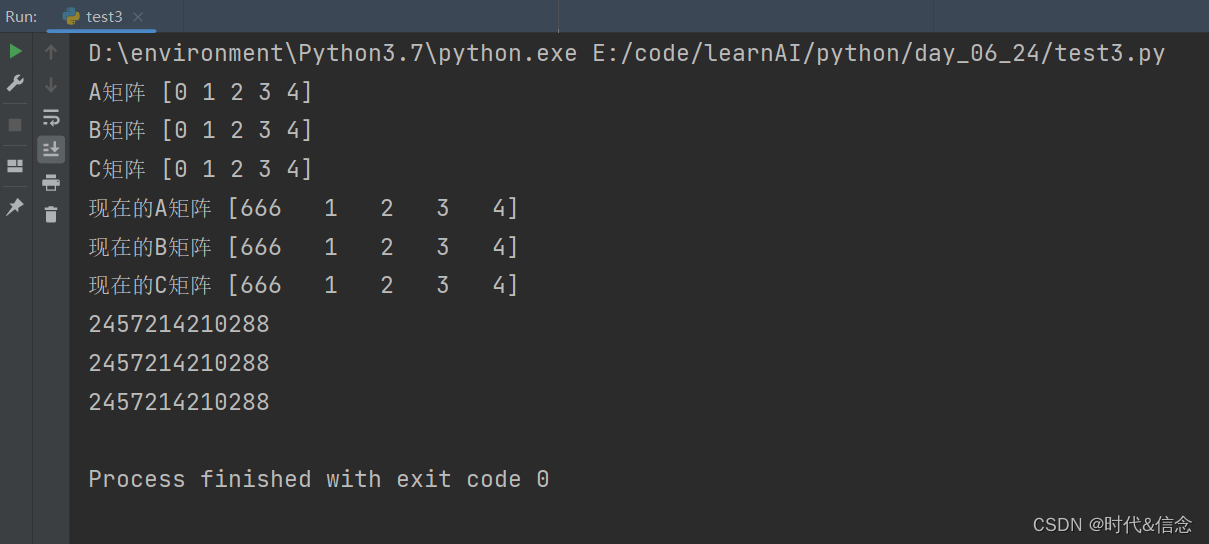#### （2）深拷贝

``````# 深度拷贝是，地址不一致， 新开辟了空间
D = A.copy()
print("A的地址是", id(A))
print("D的地址是", id(D))

print("A矩阵", A)
print("D矩阵", D)
# 改变A矩阵,深拷贝之后，他们并不公用内容，所以互不影响
A = 999
print("现在的A矩阵", A)
print("现在的D矩阵", D)
``````